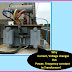We know that Current will decrease with increasing of voltage and current will increase with decreasing of voltage in the transformer but Power and Frequency always constant in a Transformer.
So there are many questions arise about it such as,
Why current decrease when voltage increase in a Transformer?
Why power does not change or constant in a Transformer?
Why frequency does not change or constant in a Transformer?
Today we are going to discuss Why Current, Voltage changes But Power, Frequency constant in Transformer?

## How a Transformer can increase or decrease the voltage?

Actually, increasing and decreasing the voltage depends on the turns ratio of the windings of the Transformer. If the no. of turns of the secondary winding is greater than the primary winding then the transformer will increase the voltage level and vice-versa.

But practically how it is possible? We know that the Transformer basically works in the principle of Faraday's Laws of Electromagnetic Induction. The magnetic flux(which changing in magnitude and direction) produced by the primary winding is linked with the secondary winding and EMF is induced in the secondary winding, thus we get the output voltage from the transformer. So if the no. of turns is more, then obviously the flux linkage with the secondary winding will be more and more EMF will be induced. Thus the Transformer increases the voltage.

## Why current decrease when voltage increase in a Transformer?

In a step-up transformer, the secondary voltage is greater than the primary voltage and secondary current is lower than the primary current.
That means if a load (connected with the step-up transformer) draws current from the transformer is I1 and Transformer draws current from the power supply is I2 then,
I1>I2
So there is a question arise why secondary current is always lower than the primary current in the step-up transformer.

When the transformer is on No-Load, it draws No-Load current I0 from the power supply and set up an MMF (N1I0) which produces flux  Ф. This produced flux linked with both winding and induced EMF in the secondary winding.

When a Load is connected across the secondary winding of the transformer, load draws current from the transformer is I2 which is flowing through the load and the secondary winding. This load current sets up an MMF ( N2I2) which produces a flux Ф2 in the secondary winding. As the secondary winding has more turns than primary winding so a large amount of flux(Ф2) will be produced.
So, Ф2

According to Lenz's Law Ф2 opposes the Ф, so the main flux Ф becomes weak and Primary EMF E1 tends to reduce. As a result, there is a difference will be created between E1 and V1. So more current drawn from the source to produced more flux in the primary winding to compensate Ф

That is why a step up transformer draws more current(I1) than load current(I2).

## Why power does not change in a Transformer?

Due to some losses such as Copper Loss, Iron Loss Input Power and Output power does not exactly the same in a Transformer. But if we think about the ideal transformer, Power does not change or Input Power is equal to Output power.

As the Increasing and decreasing of voltage and current depends upon the turns ratio of the transformer that is why Power remain constant. Yes, the current also depends upon the turns ratio which is explained in the above paragraph.

In a step-up transformer, the secondary voltage is greater than the primary voltage due to the turns ratio and the primary current is greater than the secondary current also due to the turns ratio.

In a transformer, secondary current depends on the load and the primary current depends upon the load current and turns ratio.

So, as the current decreases with increasing voltage and current increases with decreasing of voltage that is why power does not change in Transformer.

V1I1 = V2I2

## Why Frequency does not change in a Transformer?

Frequency is the no. of cycles per second. Frequency indicates how many times the magnitude and direction of voltage and current changes per second.

As the transformer is a Static device or both windings are at standstill, Frequency does not change in Transformer.

Input frequency creates a pattern in the Flux produced by the primary winding. The same flux is linked with secondary winding maintaining the same pattern. That is why the frequency of secondary winding the same as the input frequency.

Why Current, Voltage changes But Power, Frequency constant in Transformer?Reviewed by Author on March 26, 2019 Rating: 5